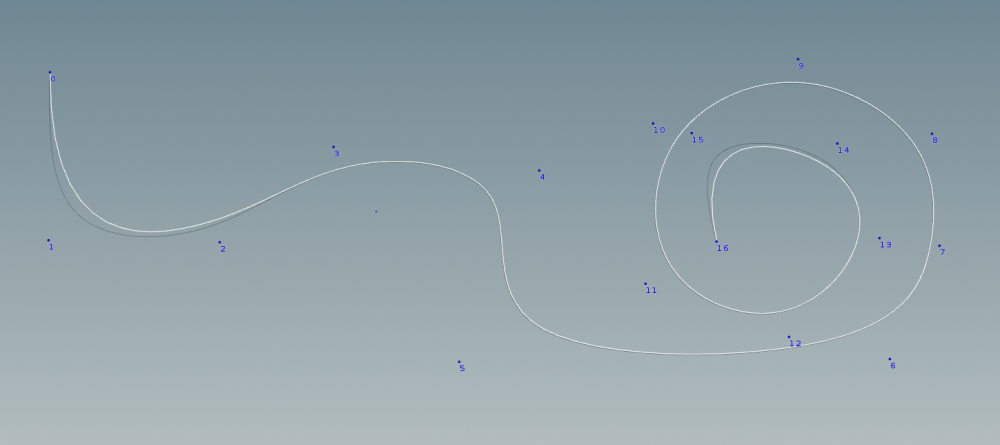# What does resample / Subdivision curves converge to ?

## Recommended Posts

Does SOP resample  / subdivision curves converge to a specific curve ?

If I use a polyline built on a NURBS control points as input, it almost converges to the original NURBS curve, except for the first and last three segments.Edited by AntoineSfx

##### Share on other sites
47 minutes ago, AntoineSfx said:

Does SOP resample  / subdivision curves converge to a specific curve ?

If I use a polyline built on a NURBS control points as input, it almost converges to the original NURBS curve, except for the first and last three segments.

I may be overlooking something here. The points are in the XY plane.

I'm not sure if a convert SOP using a polyline as input and its type set to NURBS curve is even supposed to converge to the same curve as the initial NURBS curve built on those same points, as there is also a knot vector associated with the sequence of points in each case.

Also, it is not documented  that resample  / subdiv curve will actually converge to a NURBS curve, so I don't blame them.

Some background here.. I'm trying to see if I can strategically place some points on a polyline so that it SOP resample / subdiv curve to a sequence of straight lines and circle arcs.

Circles arcs and circles can actually be represented exactly with NURBS, so that's why I'm investigating this. If I can set the right order and knot sequence, I hope it will actually converge to circle arcs and straight lines, but I'm not there yet..nurbs.hipnc

##### Share on other sites

Curve Polyline..(bezier,NUrbs) This You need OR ?

```//midPOINT
int src_prim = 0;
int num_vtx = primvertexcount(1, src_prim);
int is_prim_looped = isLooped_PrimVertex(1, src_prim);
int append_num = 0;
float append_len = 1.0;

#if (APPEND_TYPE == 0)
append_num = `chs("append_num")`;
#elif (APPEND_TYPE == 1)
append_len = `chs("append_len")`;
#endif
int num_prims = nprimitives(1);
if (0 < num_prims)
{
vector pts[];
// primitive
for (int i=0; i<num_vtx-1; ++i)
{
int vtx_curr = primvertex(1, src_prim, i);
int vtx_next = primvertex(1, src_prim, i+1);
int pt_curr  = vertexpoint(1, vtx_curr);
int pt_next  = vertexpoint(1, vtx_next);
vector pos_0 = point(1, "P", pt_curr);
vector pos_1 = point(1, "P", pt_next);
#if (APPEND_TYPE == 1)
float edge_len = distance(pos_0, pos_1);
append_num = floor(edge_len / append_len);
#endif

if ((i==0) && (is_prim_looped == 0))
{
int append_1st_num = 0;
vector first_mid_pos = lerp(pos_0, pos_1, float(1)/(append_num+1));
for (int s=0; s<append_1st_num; ++s)
{
float rate = clamp(float(s)/(append_1st_num-1), 0, 1);
vector pos = lerp(pos_0, first_mid_pos, rate);
append(pts, pos);
}
}
if (0 < append_num)
{

for (int j=0; j<append_num; ++j)
{
float rate = clamp(float(j+1)/(append_num+1), 0, 1);
vector mid_pos = lerp(pos_0, pos_1, rate);
append(pts, mid_pos);
}
}
append(pts, pos_1);
}
append_PolyLine_from_Points(pts, is_prim_looped);
}
----------------------

//EdgePOINT
int src_geo = 1;
int src_prim = 0;
int num_vtx = primvertexcount(1, src_prim);
int is_prim_looped = dgfx_IsLooped_PrimVertex(1, src_prim);
vector pts[];
int order = `chs("u_order")`;
float round_rate = `chs("round_rate")`;
dgfx_Append_Mid_Point_Edge_Array(pts, src_geo, src_prim, num_vtx, is_prim_looped, order, round_rate);
int pre_len = len(pts);
dgfx_Append_PolyLine_from_Points(pts, is_prim_looped);```Edited by Librarian

## Create an account

Register a new account